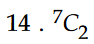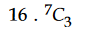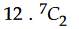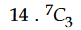#### Let A = {x1,x2,x3......,x7} and B = { y1 ,y2 ,y3 } be two sets containing seven and three distinct elements respectively. Then the total number of functions: AB that are onto, if there exist exactly three elements x in A such that(x) = y2, is equal to : Option 1)Option 2)Option 3)Option 4)As we learnt in

Number of Onto function -&Number of onto functions-

No. of onto functions.

Since if there exist exactly three elements x in A. Which give single image y2. If means four elements of A will give image of  y1 and y2. So that (24 - 2) of.Correct option is 3.

Option 1)This is an incorrect option.

Option 2)This is an incorrect option.

Option 3)This is an incorrect option.

Option 4)This is the correct option.

#### divya.saini